Get our FREE VBA eBook of the 30 most useful Excel VBA macros.

Automate Excel so that you can save time and stop doing the jobs a trained monkey could do.

# SORT function in ExcelThe SORT function is one of Excel’s best new features.  It’s one of a group of functions that make use of Excel’s new dynamic array calculation engine, enabling Excel to spill results into multiple cells from a single formula.

At the time of writing, the SORT function is only available to those on a Microsoft 365 subscription.  It will not be available in Excel 2019 or earlier versions.

I recommend you download the example file for this post.  Then you’ll be able to work along with examples and see the solution in action, plus the file will be useful for future reference.

Watch the video:

Contents

## Arguments of the SORT function

Before we look at the arguments required for the SORT function, let’s look at a basic example to appreciate what it does.

As demonstrated below, the SORT function does exactly what you would expect; it sorts the data.  The critical point is to notice that it achieves this with a single function in a single cell and spills the other results into the cells below.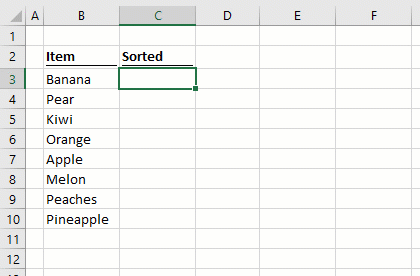SORT has four arguments:

`=SORT(array, [sort_index], [sort_order], [by_col])`
• array: The range of cells, or array of values to be sorted.
• [sort_index]: The nth column or row to apply the sort to.  For example, to sort by the 2nd column, the sort index would be 2.  It is possible to sort by multiple columns (covered in Example 6 below).  If this argument is excluded, it will default to sorting by the first column.
• [sort_order]: 1 = sort in ascending order, -1 = sort in descending order (if excluded the argument will default to 1).
• [by_col]: TRUE = sort by columns, FALSE = sort by rows (if excluded the argument will default to FALSE).## Examples of using the SORT function

The following examples illustrate how to use the SORT function.

### Example 1 – SORT returns an array of rows and columns

In this example, a single formula sorts the values in the first column and returns the full range of cells provided by the array argument.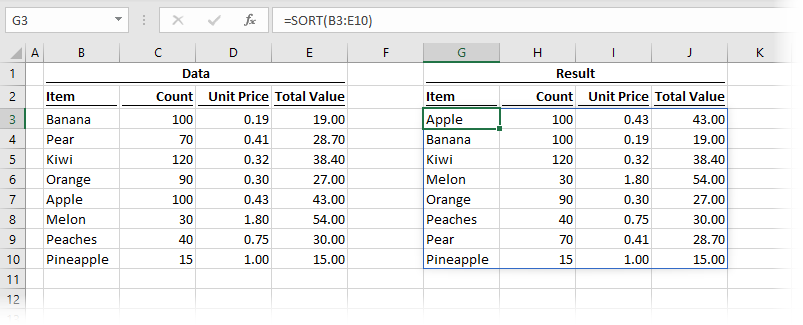The formula in cell G3 is:

`=SORT(B3:E10)`

This single formula is returning eight rows and four columns of data.  As the second, third, and fourth arguments have been excluded, the default has been applied for each of them, sorting by the first column, in ascending order with data organized in rows.

### Example 2 – SORT by another column in descending order

Example 2 shows how to sort by the second column in descending order.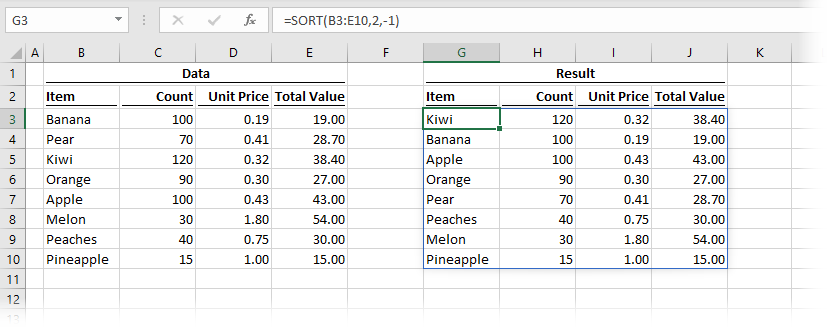The formula in cell G3 is:

`=SORT(B3:E10,2,-1)`

The second argument in the SORT function is the sort_index.  The formula above is sorting by the 2nd column of the array.

The third argument is the sort_order.  The -1 in this formula denotes the data sorts in descending order.

### Example 3 – SORT expands automatically when linked to a table

The animation below shows how the SORT function responds when linked to an Excel table.In this example, the SORT function is using an Excel table as its source data array.  New records added are automatically added to the spill range of the function.

### Example 4 – Using SORT to return a top 5 and specific columns

Example 4 shows how to create a top 5 and select which specific columns to return.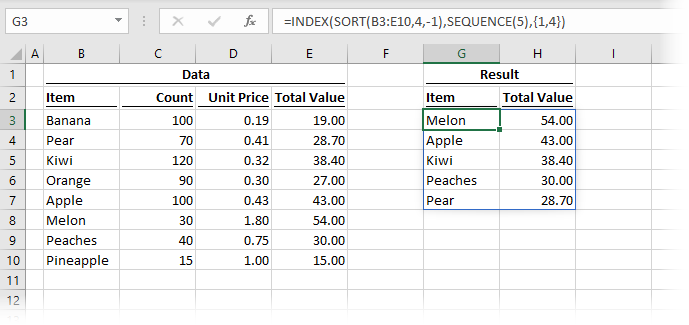The formula in cell G3 is:

`=INDEX(SORT(B3:E10,4,-1),SEQUENCE(5),{1,4})`

We are using two additional functions in this example, SEQUENCE (also a new function) and INDEX (which is not new, it has been around forever).

The SORT is applied to the 4th column in descending order, we have seen similar examples to this above.

INDEX is taking the result of the SORT function and using:

• The SEQUENCE function to only show the first 5 results
• A constant array to display only columns 1 and 4.

In the past, this would have needed a lot of calculations, but now it’s possible with a single function – Amazing!

### Example 5 – Combining FILTER and SORT

The dynamic array functions can be nested within each other.  This example shows the FILTER function nested inside SORT.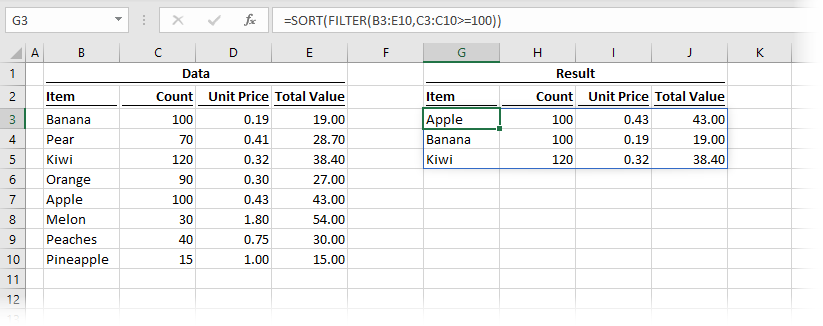The formula in cell G3 is:

`=SORT(FILTER(B3:E10,C3:C10>=100))`

The FILTER function is returning only three rows, where the values in cells C3-C10 are 100 or higher.  The SORT is then applied to the result of the FILTER, to provide those filtered rows in alphabetical order.

### Example 6 – SORT on multiple columns

SORT can be applied to multiple columns at the same time.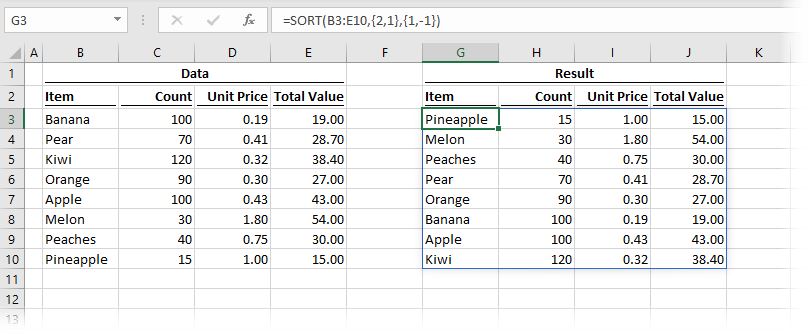The formula in cell G3 is:

`=SORT(B3:E10,{2,1},{1,-1})`

This formula contains two constant arrays.   The first, {2,1} is the sort_order, which in this example is sorting by column 2 then by column 1.  The second constant array is {1,-1}, which determines how each column sorts.  The first sort (applied to column 2) is in ascending order, and the second sort (applied on column 1) is in descending order.

There is a lot to learn about dynamic arrays and the new functions.  Check out my other posts here to learn more:Get our FREE VBA eBook of the 30 most useful Excel VBA macros.

Automate Excel so that you can save time and stop doing the jobs a trained monkey could do.

Don’t forget: